TOP PAGE

# Learning technique

## Ordinary learning

Learning results of the value network in Chapter 10.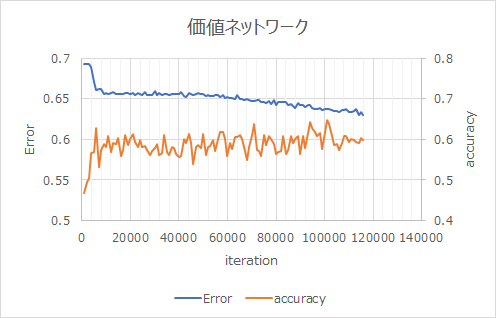## Transfer learning

Learning by transferring the learning results of the policy network. The error was low and the accuracy was high.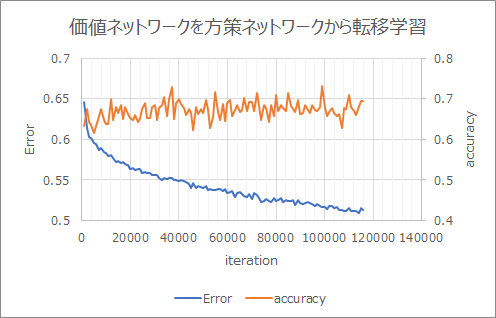The upper row is Chapter 7 Policy Network The bottom row is Chapter 10 Value Network The idea of multitask learning is to make it common because the light blue parts are the same.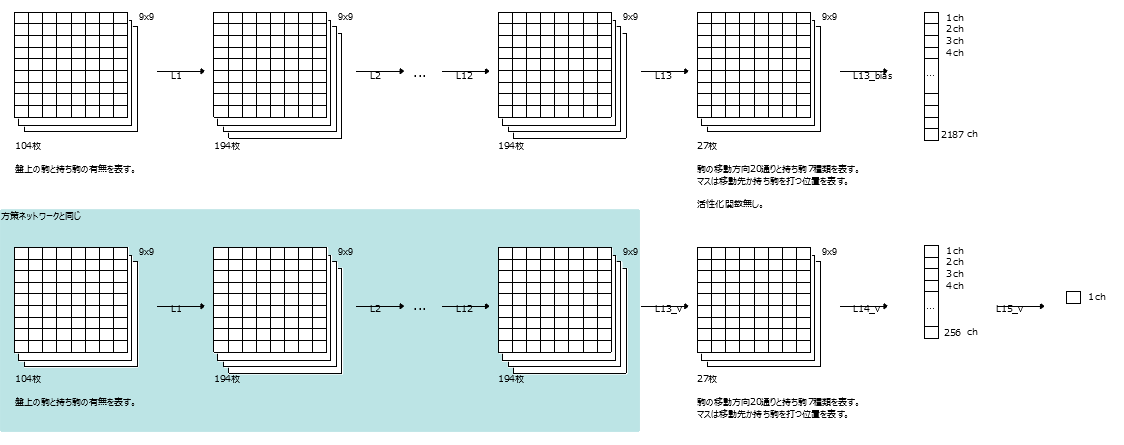This is what happens when the light blue is standardized.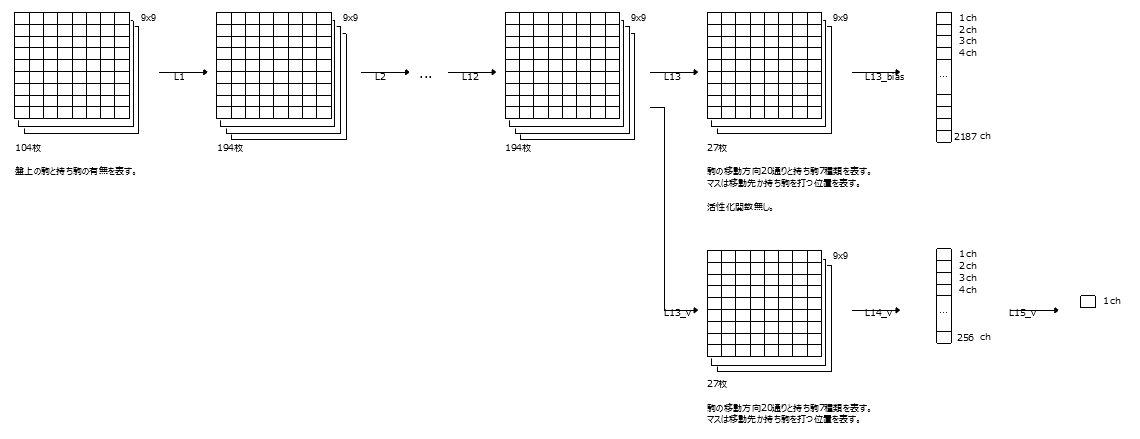You can learn policies and values at the same time. The accuracy is also good.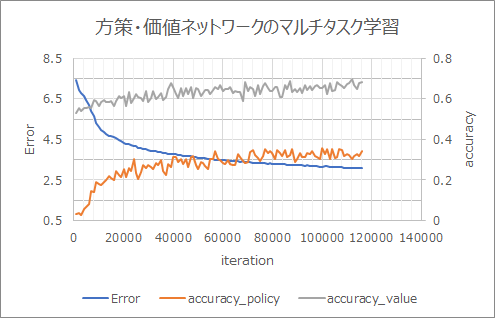Residual Network It seems that the configuration called ResNet is good. I don't understand why ResNet is good, but it seems that research is progressing.

1 block of ResNet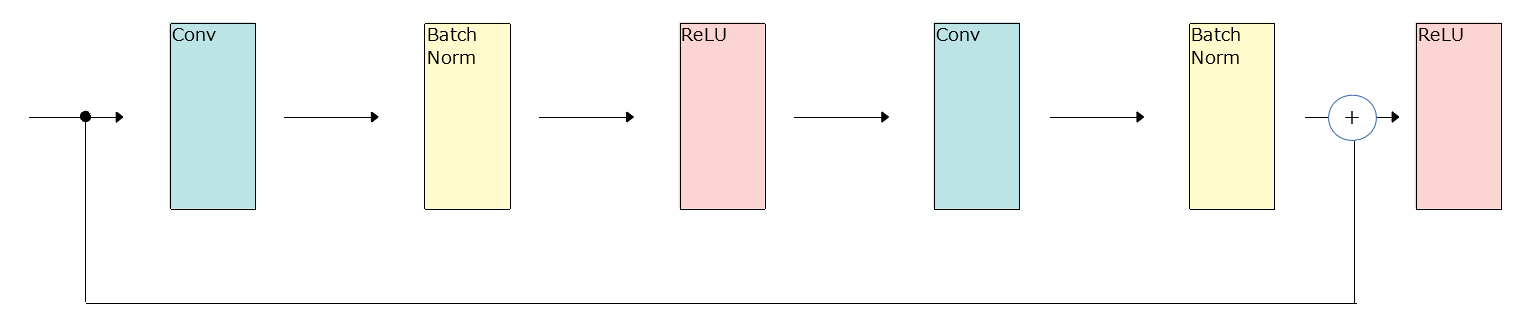Details of one block of ResNet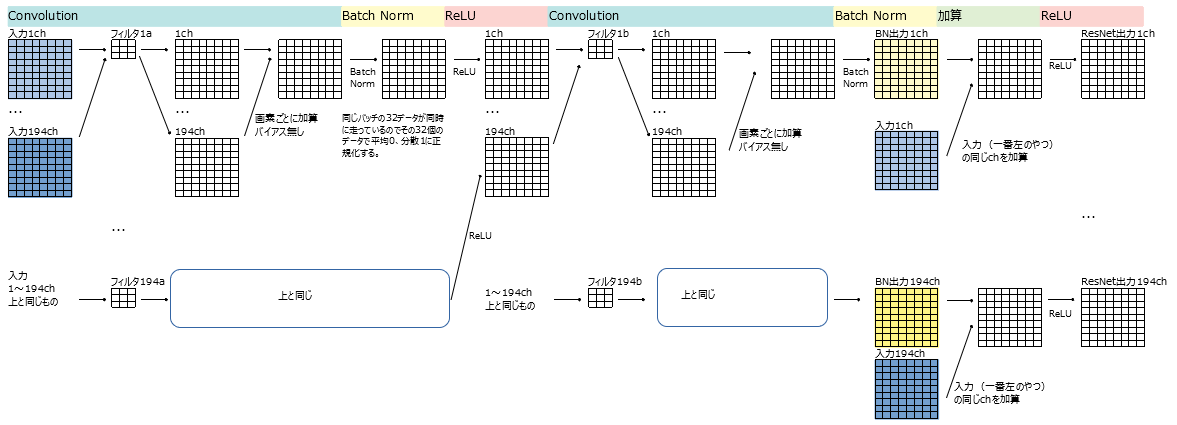Connect 5 ResNet blocks and replace them with L2 to L12 for multitask learning.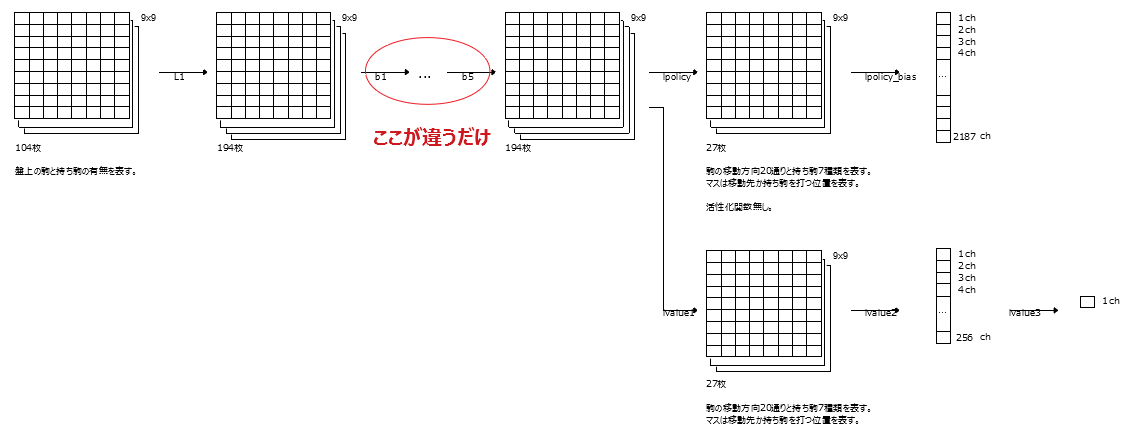Learning results Learning is progressing faster than without ResNet.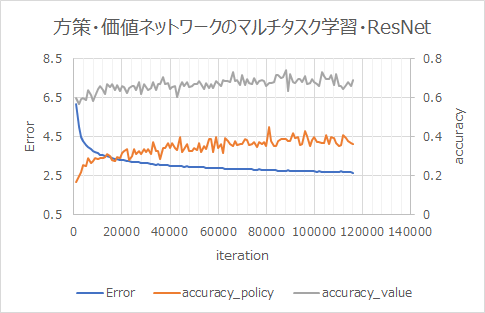policy_value_resnet.py

x + h2 x + h2 is the operation of adding x and h2 "element by element". (When I actually printed the value, it was like that)

``````    def __call__(self, x):
h1 = F.relu(self.bn1(self.conv1(x)))
h2 = self.bn2(self.conv2(h1))
return F.relu(x + h2)
``````

h = self['b{}'.format(i)]（h） This way of writing means self.bi (h).

``````        for i in range(1, self.blocks + 1):
h = self['b{}'.format(i)](h)
``````

#### `pydlshogi/network/policy_value_resnet.py`

``````
#!/usr/bin/env python3
# -*- coding: utf-8 -*-

from chainer import Chain
import chainer.functions as F

from pydlshogi.common import *

ch = 192
fcl = 256

class Block(Chain):
def __init__(self):
super(Block, self).__init__()
with self.init_scope():
self.conv1 = L.Convolution2D(in_channels = ch, out_channels = ch, ksize = 3, pad = 1, nobias=True)
self.bn1   = L.BatchNormalization(ch)
self.conv2 = L.Convolution2D(in_channels = ch, out_channels = ch, ksize = 3, pad = 1, nobias=True)
self.bn2   = L.BatchNormalization(ch)

def __call__(self, x):
h1 = F.relu(self.bn1(self.conv1(x)))
h2 = self.bn2(self.conv2(h1))
return F.relu(x + h2)
# x +When h2 printed the value and confirmed it, x and h2 were added element by element. In other words, x, h2, x + h2 are 194 elements.
#I was worried that the first feeling would be 388 elements, so I checked it, but F.Does it mean that each element is added in the relu?

class PolicyValueResnet(Chain):
def __init__(self, blocks):
super(PolicyValueResnet, self).__init__()
self.blocks = blocks
with self.init_scope():
self.l1 = L.Convolution2D(in_channels = 104, out_channels = ch, ksize = 3, pad = 1)
for i in range(1, blocks + 1):
self.add_link('b{}'.format(i), Block()) #The first argument is the name and the second argument is the class
# policy network
self.lpolicy = L.Convolution2D(in_channels = ch, out_channels = MOVE_DIRECTION_LABEL_NUM, ksize = 1, nobias = True)
self.lpolicy_bias = L.Bias(shape=(9*9*MOVE_DIRECTION_LABEL_NUM))
# value network
self.lvalue1 = L.Convolution2D(in_channels = ch, out_channels = MOVE_DIRECTION_LABEL_NUM, ksize = 1)
self.lvalue2 = L.Linear(9*9*MOVE_DIRECTION_LABEL_NUM, fcl)
self.lvalue3 = L.Linear(fcl, 1)

def __call__(self, x):
h = F.relu(self.l1(x))
for i in range(1, self.blocks + 1):
h = self['b{}'.format(i)](h) #This way of writing self.b How many.
# policy network
h_policy = self.lpolicy(h)
policy = self.lpolicy_bias(F.reshape(h_policy, (-1, 9*9*MOVE_DIRECTION_LABEL_NUM)))
# value network
h_value = F.relu(self.lvalue1(h))
h_value = F.relu(self.lvalue2(h_value))
value = self.lvalue3(h_value)
return policy, value

``````